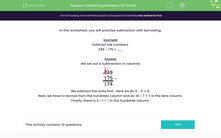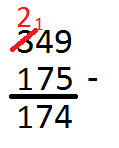# Subtract Three-Digit Numbers Using Borrowing

In this worksheet, students will practise formal written methods of subtraction for calculations involving two three-digit numbers and one three-digit and one two-digit number.Key stage:  KS 2

Curriculum topic:   Number: Addition and Subtraction

Curriculum subtopic:   Add/Subtract up to Four-Digit Numbers

Difficulty level:#### Worksheet Overview

In this activity, we will be practising subtraction with borrowing.

Example

Subtract the numbers.

349 - 175 = __

We set out a subtraction in columns

.We subtract the ones first.  Here we do 9 - 5 = 4.

Next, we have to borrow from the hundreds column and do 14 - 7 = 7 in the tens column.

Finally, there is 2 - 1 = 1 in the hundreds column.

Are you happy with all that?Let's get started on the questions. If you need a reminder of how to do borrowing, just click on the red help button that will appear on the screen when you start the questions. It will show you this page again.

Let's go!

### What is EdPlace?

We're your National Curriculum aligned online education content provider helping each child succeed in English, maths and science from year 1 to GCSE. With an EdPlace account you’ll be able to track and measure progress, helping each child achieve their best. We build confidence and attainment by personalising each child’s learning at a level that suits them.

Get started